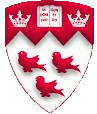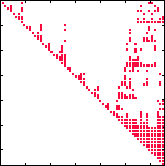McGill University 189-578A Introduction to Scientific Computing  (4 credits) Professor: Martin J. Gander### Course Description

Most real life problems can not be solved exactly by analytical tools.Their solution has to be approximated using numerical techniques. This course gives a broad introduction to the field of scientific computing with focus on problem solving using modern tools. We use throughout the course Maple for symbolic calculations and Matlab to develop numerical codes. The goal of the course is to get fundamental knowledge of the underlying numerical methods in black box solvers and thus to be able to choose and use them with confidence and interpret the obtained results. This is the ideal course for you if you are an applied mathematician or engineer who wants to understand the numerical methods you are using and make an informed choice about methods. After the course you will be able to understand problems in your calculations and deal with them.

### Topics

• Is error analysis and computer arithmetic in finite precision that important ?
• Nonlinear equations: bisection, Newtons method and its variants and fixed point methods.
• Linear systems: direct factorizations, stationary iterative methods (Jacobi, Gauss-Seidel, SOR) and Krylov subspace methods (Conjugate Gradient, GMRES).
• Eigenvalue problems: Jacobi, power iterations, the QR algorithm and Lanczos.
• Interpolation: Polynomials, splines and geometric interpolation.
• Approximation: linear and nonlinear least squares (normal equations, QR decomposition).
• Differentiation: finite differences and Richardson extrapolation.